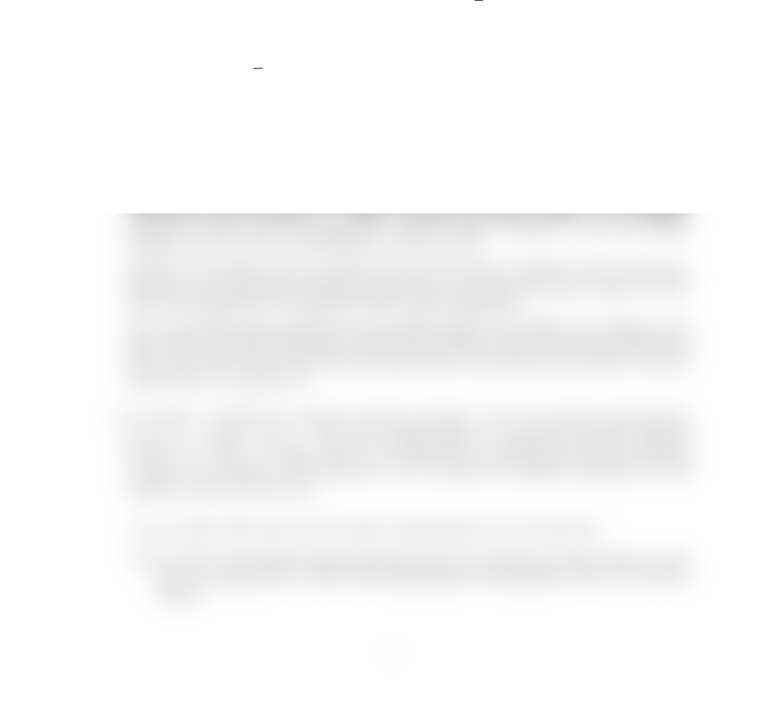# Assignment #2 This is the second assignment from winter 2010 term

40 views2 pages
School
CourseCS 341, Winter 2010
Timothy Chan
Assignment 2 (due February 11 Thursday noon)
structions.
1. [10 marks] Consider the following recurrence:
T(n) =
(
T(n/2) + 2 T(n/4) + nif n > 2
1 if n2
Use the guess-and-check (substitution) method to prove the upper bound T(n) = O(n). Hint:
use T(n)cn c0nas the guess, where cand c0are suitable constants. You may assume
that nis a power of 2.
2. [14 marks] Given an array of nelements A,...,A[n] where nis even, we want to swap
elements so that at the end the array becomes
A, A[n/2 + 1], A, A[n/2 + 2],...,A[n/2], A[n].
Call this the shuﬄe problem (if we think of the array as a deck of cards, we are essentially
splitting the deck evenly and performing a perfect shuﬄe). Example: if the array initially
contains 1,2,3,4,5,6,7,8, it will become 1,5,2,6,3,7,4,8.
Obviously, the problem can be solved in O(n) time by using a temporary array of O(n) size.
However, we are interested in algorithms that do not use much extra space besides the given
array (such algorithms are sometimes called in-place algorithms).
Give an O(nlog n)-time algorithm for the shuﬄe problem that requires only O(log n) extra
space. Hint: use divide-and-conquer; besides local variables, the only extra space should come
from the recursion stack, which has size proportional to the depth of the recursion. You may
assume that nis a power of 2.
3. [24 marks] Consider the decimal conversion problem: given the decimal representation
dn1dn2···d0(di∈ {0,1,...,9}) of an n-digit number N, compute the binary represen-
tation of N. Here, nis large, and we are interested in running times that are measured
in terms of the number of bit operations. We will explore two diﬀerent algorithms for this
problem (in parts (b) and (d)).
(a) [2 marks] First show that the binary representation of Nhas O(n) bits.
(b) [6 marks] The following simple algorithm solves the decimal conversion problem. Show
that its running time is O(n2) (the multiplications and additions in line 3 are done in
binary):
1
Unlock document

This preview shows half of the first page of the document.
Unlock all 2 pages and 3 million more documents.

# Get access

\$10 USD/m
Billed \$120 USD annually
Homework Help
Class Notes
Textbook Notes